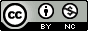## Tuesday, June 16, 2009

### NYT: In Simulation Work, the Demand Is Real

The New York Times published this interesting article on how the ability to design and perform computer simulations is a highly marketable skill for careers across many disciplines.

In methodology development we use simulation nearly every day. We've developed our own specialized genetic data simulation software, genomeSIMLA, that's freely available here by request for PC, Mac, and Linux.

But if you have R on your computer (get it free here), here's how to do a really simple Monte Carlo simulation to determine the power of a one-sample t-test.

First, fire up R and type this command:

rnorm(100)

That command generates 100 random numbers drawn from a standard normal distribution, mean=0, sd=1. Now type this:

rnorm(100,mean=2,sd=7)

That also draws 100 random numbers from a normal distribution, but this time the mean is 2 and the standard deviation is 7. You can also get the same results by just typing this:

rnorm(100,2,7)

Now, let's do a one sample t-test:

t.test(rnorm(100,2,7))

That command performs a one-sample t-test on the 100 samples drawn from a normal distribution with mean=2 and sd=7. Remember, the null hypothesis of a one-sample t-test is usually "the mean is not significantly different from zero". So if the p-value is less than .05, we would typically reject this null, and say that the mean is significantly different from zero.

Now, we knew that the mean was different from zero, because we said draw from a distribution with mean=2. But if this was the case and we only drew 100 samples, how likely is it that we would detect a difference? That's the power of the test - given that the null is false, how likely is it that we reject the null hypothesis?

One way we can answer this question is with a simulation.

First, let's type the same command, but just get ONLY the p-value from the t-test:

t.test(rnorm(100,2,7))\$p.value

Was is less than .05? Try typing it again (you can hit the up arrow key to bring up the last command in R, just like on the Linux command line). It will be different because we have a different set of 100 observations. Type it in over and over again. Sometimes it will be less than .05, other times it wont be. Let's do this 1000 times, and see how often it is less than .o5. Let's use the replicate command:

replicate(1000,t.test(rnorm(100,2,7))\$p.value)

That simulates doing the t-test 1000 times, and gives you the p-value from each one.

Now, let's do a logical test to see which of those are less than .05:

replicate(1000,t.test(rnorm(100,2,7))\$p.value)<0.05

If you typed that in you'll see lots of TRUE's and FALSE's. TRUE means that the t-test on that particular sample was less than .05. Now, internally, R represents TRUE as 1, and FALSE as 0. So if we take the average of all 1000 of these, that will tell us the proportion of times out of 1000 trials that the p-value of the one-sample t-test was less than .o5:

mean(replicate(1000,t.test(rnorm(100,2,7))\$p.value)<0.05)

When I did this the power was right around 80%. If you do this again it will be slightly different because remember we are sampling randomly so the results will vary slightly!

Congratulations, you just did your first simulation / power study! Of course because we know what the null distribution of t-statistics looks like under the null, we can mathematically determine the power of a t-test without doing simulation studies:

power.t.test(n=100,delta=2,sd=7,sig.level=.05,type="one.sample")

But if we had developed our own method or algorithm we probably wouldn't have a mathematical formula to calculate power, which is why we rely on simulation studies. Be sure to check out my other posts on power calculation software, choosing the correct analyses, and code to run analyses in R and other software.